# Knapsack problem

(diff) ← Older revision | Latest revision (diff) | Newer revision → (diff)

Given a knapsack (container) of total capacity, andobjects with weights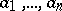and respective values, the problem is to pack as much value in the knapsack as possible.

Abstractly the problem can be formulated as follows. Given positive integers,,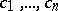, the problem is to maximizesubject to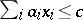and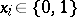.

The greedy algorithm to "solve" this proceeds as follows. It is natural to favour objects with the greatest value/weight density. So, relabel, if needed, the objects so that. Then selectrecursively according toThis greedy algorithm has a performance ratio of, i.e. it is guaranteed to find a solution which is within a factorof the optimal one.

If the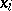are allowed to take fractional values (i.e. values in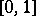instead of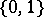), then the greedy algorithm is optimal.

The knapsack problem is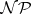-hard, cf..

How to Cite This Entry:
Knapsack problem. Encyclopedia of Mathematics. URL: http://encyclopediaofmath.org/index.php?title=Knapsack_problem&oldid=16554
This article was adapted from an original article by M. Hazewinkel (originator), which appeared in Encyclopedia of Mathematics - ISBN 1402006098. See original article